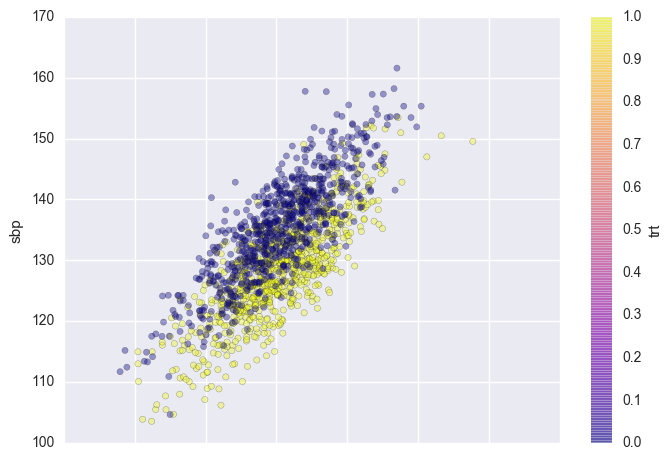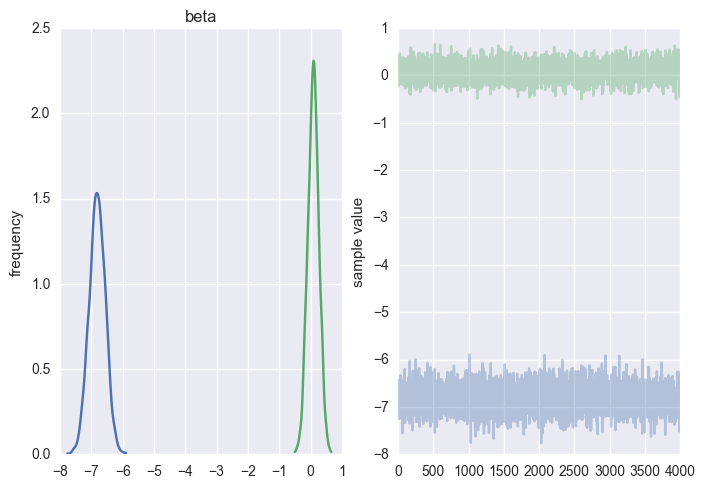# Simulated clinical trial¶

Simulated randomized clinical trials (RCT) are useful because we know the true treatment effects being estimated. Consider a two-treatment (A, B) RCT for hypertension where covariate adjustment is used for baseline systolic blood pressure (SBP) and where there are two outcomes: (1) incidence of death or stroke (DS) within one year and (2) systolic blood pressure at one year after randomization. Even though time to DS would be a preferred outcome (and would handle censoring) we ignore the timing of events for this example and use a binary logistic model for DS. SBP is assumed to follow a normal distribution with constant SD $\sigma=7$ given baseline SBP. The true B:A treatment effect is assumed to be a mean 7mmHg drop in SBP with B. We assume a correlation between SBP reduction and incidence of DS by forming a population logistic model for DS in which baseline and 1y SBP each have a regression coefficient of 0.05 for predicting DS and treatment has a coefficient of log(0.8). To estimate the true effect of treatment on DS not adjusted for follow-up SBP we first simulate a trial with $n=40000$.

The regression coefficient for treatment in the proper outcome model that did not adjust for 1y SBP was round(btrt, 4) which corresponds to a B:A odds ratio of round(exp(btrt), 4) which we take as the true treatment effect on the binary outcome.

The trial could easily be run sequentially but we treat the sample size as fixed at $n=1500$ and simulate the trial data as such.

In :
%matplotlib inline
import numpy as np
import pandas as pd
import matplotlib.pyplot as plt
import seaborn as sns
sns.set()
import pystan
import statsmodels.api as sm
import statsmodels.formula.api as smf
from scipy.stats import logistic

np.random.seed(1)

/Users/fonnescj/anaconda3/envs/dev/lib/python3.5/site-packages/Cython/Distutils/old_build_ext.py:30: UserWarning: Cython.Distutils.old_build_ext does not properly handle dependencies and is deprecated.
"Cython.Distutils.old_build_ext does not properly handle dependencies "

In :
invlogit = lambda x: 1 / (1 + np.exp(-x))

In :
A,B = 0,1

def sim(n):
trt = np.r_[np.ones(int(n/2)), np.zeros(int(n/2))]
sbp0 = np.random.normal(140, 7, size=n)
sbp = sbp0 - 5 - 7*(trt==B) + np.random.normal(scale=5, size=n)
logit = -2.6 - np.log(0.8)*(trt==B) + 0.05*(sbp0 - 140) + 0.05*(sbp - 130)
ds = np.arange(2)[(np.random.random(n) <= invlogit(logit)).astype(int)]

df = pd.DataFrame({'trt': trt,
'sbp0': sbp0,
'sbp': sbp,
'ds': ds})
return df

In :
n = 1500
data = sim(n)
data.plot.scatter('sbp0', 'sbp', c='trt', cmap='plasma', alpha=0.4);In :
fit1 = smf.ols(formula='sbp ~ sbp0 + trt', data=data).fit()
fit1.summary()

Out:
Dep. Variable: R-squared: sbp 0.712 OLS 0.712 Least Squares 1852. Mon, 07 Nov 2016 0.00 17:14:18 -4532.6 1500 9071. 1497 9087. 2 nonrobust
coef std err t P>|t| [95.0% Conf. Int.] -4.8409 2.595 -1.865 0.062 -9.932 0.250 0.9978 0.018 54.137 0.000 0.962 1.034 -6.9345 0.257 -27.007 0.000 -7.438 -6.431
 Omnibus: Durbin-Watson: 2.361 2.015 0.307 2.373 0.071 0.305 2.866 2840
In :
fit2 = smf.glm(formula='ds ~ sbp0 + sbp + trt', data=data, family=sm.families.Binomial()).fit()
fit2.summary()

Out:
Dep. Variable: No. Observations: ds 1500 GLM 1496 Binomial 3 logit 1.0 IRLS -461.49 Mon, 07 Nov 2016 922.98 17:14:18 1.50e+03 8
coef std err z P>|z| [95.0% Conf. Int.] -15.7563 1.922 -8.197 0.000 -19.524 -11.989 0.0784 0.022 3.572 0.000 0.035 0.121 0.0173 0.018 0.983 0.325 -0.017 0.052 0.2147 0.215 0.998 0.318 -0.207 0.636

Specify Stan model

In :
model = """
data {
int n;
vector[n] x;
real y1[n];
int y2[n];
vector[n] treat;
vector<lower=0> sigma_b;
vector Zero;
}

parameters {
vector alpha;
vector beta;
vector mu;
real<lower=0> sigma;
corr_matrix Omega_b;
}

transformed parameters {
vector[n] theta1;
vector[n] theta2;
cov_matrix Sigma;

Sigma <- quad_form_diag(Omega_b, sigma_b);

theta1 <- mu + alpha*x + beta*treat;
theta2 <- mu + alpha*x + beta*treat;
}

model {
beta ~ multi_normal(Zero, Sigma);
Omega_b ~ lkj_corr(1);

sigma ~ cauchy(0, 5);

y1 ~ normal(theta1, sigma);
y2 ~ bernoulli_logit(theta2);
}

generated quantities {
real A;
real B;
real A_or_B;
real A_and_B;

A <- if_else(beta < -7, 1, 0);
B <- if_else(exp(beta) < 0.8, 1, 0);

A_or_B <- if_else(A || B, 1, 0);
A_and_B <- if_else(A && B, 1, 0);
}
"""

In :
fit = pystan.stan(model_code=model,
data=dict(x=data.sbp0,
y1=data.sbp,
y2=data.ds,
treat=data.trt, n=n, sigma_b=(2, 1.5), Zero=(0,0)))

INFO:pystan:COMPILING THE C++ CODE FOR MODEL anon_model_456ec81631c7e21b1d5f3580dbc6353b NOW.

In :
fit['beta'].mean(0)

Out:
array([-6.8113808 ,  0.09079104])
In :
np.exp(fit['beta'][:,1]).mean()

Out:
1.1123857364294381
In :
fit.plot(pars=['beta']);Probabilities of A OR B and A AND B::

In :
sample = fit.extract(pars=['A_or_B', 'A_and_B'])

In :
[sample[s].mean(0) for s in sample]

Out:
[0.25574999999999998, 0.0082500000000000004]

Correlation between betas

In :
fit['Omega_b'].mean(0)

Out:
array([[ 1.        , -0.01236086],
[-0.01236086,  1.        ]])